# AC, DC and Electrical Signals

Next Page: Oscilloscopes (CROs)

Also See: Diodes | Power Supplies

AC means Alternating Current and DC means Direct Current. AC and DC are also used when referring to voltages and electrical signals which are not currents! For example: a 12V AC power supply has an alternating voltage (which will make an alternating current flow).

An electrical signal is a voltage or current which conveys information, usually it means a voltage. The term can be used for any voltage or current in a circuit.

## Alternating Current (AC)

Alternating Current (AC) flows one way, then the other way, continually reversing direction.

An AC voltage is continually changing between positive (+) and negative (-).

The rate of changing direction is called the frequency of the AC and it is measured in hertz (Hz) which is the number of forwards-backwards cycles per second.

Mains electricity in the UK has a frequency of 50Hz.

See below for more details of signal properties.

An AC supply is suitable for powering some devices such as lamps and heaters but almost all electronic circuits require a steady DC supply (see below).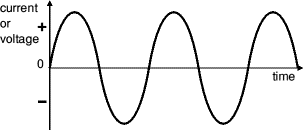AC from a power supply
This shape is called a sine wave.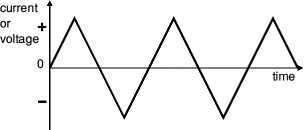This triangular signal is AC because it changes
between positive (+) and negative (-).

## Direct Current (DC)

Direct Current (DC) always flows in the same direction, but it may increase and decrease.

A DC voltage is always positive (or always negative), but it may increase and decrease.

Electronic circuits normally require a steady DC supply which is constant at one value or a smooth DC supply which has only a small variation called ripple.

Cells, batteries and regulated power supplies provide steady DC which is ideal for electronic circuits.

Power supplies contain a transformer which converts the mains AC supply to a safe low voltage AC. Then the AC is converted to DC by a bridge rectifier but the output is varying DC which is unsuitable for electronic circuits.

Some power supplies include a capacitor to provide smooth DC which is suitable for less-sensitive electronic circuits, including most of the projects on this website.

Lamps, heaters and motors will work with any DC supply.

Please see the power supplies page for further information.

Power supplies are also covered by the Electronics in Meccano website.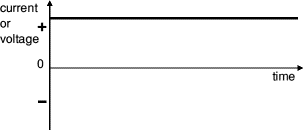from a battery or regulated power supply,
this is ideal for electronic circuits.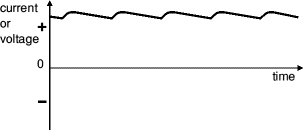Smooth DC
from a smoothed power supply,
this is suitable for some electronics.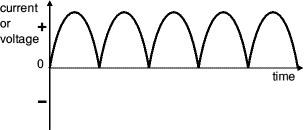Varying DC
from a power supply without smoothing,
this is not suitable for electronics.

## Properties of electrical signals

An electrical signal is a voltage or current which conveys information, usually it means a voltage. The term can be used for any voltage or current in a circuit.

The voltage-time graph below shows various properties of an electrical signal. In addition to the properties labelled on the graph, there is frequency which is the number of cycles per second.

The diagram shows a sine wave but the properties apply to any signal with a constantly repeating shape.

• Amplitude is the maximum voltage reached by the signal. It is measured in volts, V.
• Peak voltage is another name for amplitude.
• Peak-peak voltage is twice the peak voltage (amplitude). When reading an oscilloscope trace it is usual to measure peak-peak voltage.
• Time period is the time taken for the signal to complete one cycle. It is measured in seconds (s) but time periods tend to be short so milliseconds (ms) and microseconds (µs) are often used.
1ms = 0.001s and 1µs = 0.000001s.
• Frequency is the number of cycles per second. It is measured in hertz (Hz), but frequencies tend to be high so kilohertz (kHz) and megahertz (MHz) are often used.
1kHz = 1000Hz and 1MHz = 1000000Hz.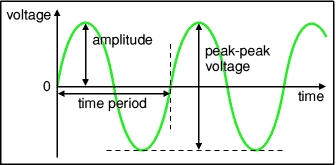#### Frequency and time period

Frequency and time period are the inverse of each other:

 frequency  = 1 time period

and

 time period  = 1 frequency

Mains electricity in the UK has a frequency of 50Hz so it has a time period of 1/50 = 0.02s = 20ms.

## Root Mean Square (RMS) Values

The value of an AC voltage is continually changing from zero up to the positive peak, through zero to the negative peak and back to zero again. Clearly for most of the time it is less than the peak voltage, so this is not a good measure of its real effect.

Instead we use the root mean square voltage (VRMS) which is 0.7 of the peak voltage (Vpeak):

 VRMS = 0.7 × Vpeak

and

 Vpeak = 1.4 × VRMS

These equations also apply to current.

It is important to note that these equations are only true for sine waves (the most common type of AC) because the 0.7 and 1.4 factors are different values for other shapes.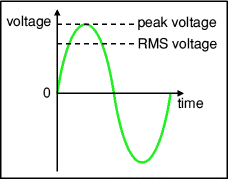The RMS value is the effective value of a varying voltage or current. It is the equivalent steady DC value which gives the same effect.

For example a lamp connected to a 6V RMS AC supply will light with the same brightness when connected to a steady 6V DC supply. However, the lamp will be dimmer if connected to a 6V peak AC supply because the RMS value of this is only 4.2V (it is equivalent to a steady 4.2V DC).

You may find it helps to think of the RMS value as a sort of average, but please remember that it is NOT really the average! In fact the average voltage (or current) of a typical AC signal is zero because the positive and negative parts exactly cancel out.

#### What do AC meters show, is it the RMS or peak voltage?

AC voltmeters and ammeters show the RMS value of the voltage or current.

#### What does '6V AC' really mean, is it the RMS or peak voltage?

If the peak value is meant it should be clearly stated, otherwise assume it is the RMS value. In everyday use AC voltages (and currents) are always given as RMS values because this allows a sensible comparison to be made with steady DC voltages (and currents), such as from a battery.

For example a '6V AC supply' means 6V RMS, the peak voltage is 8.4V. The UK mains supply is 230V AC, this means 230V RMS so the peak voltage of the mains is about 320V.

#### So what does root mean square (RMS) really mean?

First square all the values, then find the average (mean) of these square values over a complete cycle, and find the square root of this average. That is the RMS value. Confused? Ignore the maths (it looks more complicated than it really is), just accept that RMS values for voltage and current are a much more useful quantity than peak values.

Next Page: Oscilloscopes (CROs) | Study